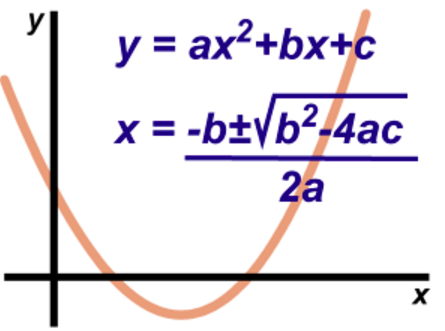3 years ago
kschneidere116
Save
Edit
Host a game
Live GameLive
Homework
Solo Practice
Practice20 QuestionsShow answers
• Question 1
120 seconds
Q. What is the discriminant?
b²-4ac
b2/2a
4ac
b2±4ac
• Question 2
120 seconds
Q. If the discriminant is negative, then the quadratic has:
1 Real Solution
2 Real Solutions
Half a Solution
No Real Solutions
• Question 3
120 seconds
Q. If the discriminant equals 0, then the quadratic has:
1 Real Solution
2 Real Solutions
Half a Solution
No Real Solution
• Question 4
120 seconds
Q. If the discriminant is positive, then the quadratic has:
1 Real Solution
2 Real Solutions
Half a Solution
No Real Solution
• Question 5
120 secondsQ. What is this?
This is the speed of light formula.
This is the zero product property.
This is scary.
• Question 6
120 seconds
Q. What should you do first in solving this equation?
x2 + 6x - 13 = 3
Get factored form
Write down: a=1, b=6, c=-13
Make it equal 0 by subtracting 3 on each side
Type it all in a calculator.
• Question 7
300 secondsQ.
√3
±√3
-3
No real solution
• Question 8
300 secondsQ.
±81
±9
±3
No real solution
• Question 9
300 secondsQ.
-4, 2
-2, 4
-16
-4
• Question 10
300 secondsQ.
-2, 4
-8
±8
No real solution
• Question 11
300 secondsQ.
±1.5
1.5
±1
No real solution
• Question 12
300 seconds
Q. If the graph of a quadratic does not intercept the x axis at any point, then it has:
1 Real Solution
2 Real Solutions
Half a Solution
No Real Solution
• Question 13
300 seconds
Q. Convert x2-6x+9 to factored form and identify the solution.
(x-3)(x-3); x=3
(x+3)(x+3); x=-3
(x+4)(x+2); x=-4, -2
Not factorable
• Question 14
300 seconds
Q. Solve     4x2 + 12x + 11 = 0
-2.2 or -0.8
-2 or -1
2 or 1
No Solution
• Question 15
300 seconds
Q. Solve    2x2 + 7x - 15 = 0
-1.5 or 5
No Solution
-5 or 1.5
0.7 or 5
• Question 16
300 secondsQ. Find the zeros of this quadratic.
b=4/5, b=3
b=4, b=-3
b=1/5, b=3
b=4/5, b=-3
• Question 17
300 secondsQ. Find the zeros.
0 and 4
0 and -4
0
-4
• Question 18
300 seconds
Q. Determine the values of
a, b, and c for
4x2 – 8x = 3
a = 4, b = -8, c = 3
a = 4, b =-8, c =-3
a = 4, b = 8, c = 3
a = 4, b = 8, c = -3
• Question 19
300 seconds
Q. Suzie solves the equation below:
x2 + 4x - 12 = 2
(x - 2)(x + 6) = 2,
by setting x -2 = 0 and x + 6 = 0.
Her solutions are x = 2 and x = -6.
Is Suzie correct? Why or why not?
Yes, she factored correctly and then used the zero product property
Yes, she factored correctly and then square rooted.
No, she did factor correctly, but she can't use the zero product property as shown.
No, she didn't factor correctly so the rest of her work is wrong.
• Question 20
300 seconds
Q. Solve by factoring.
-4y2 + 24y - 36 = 0